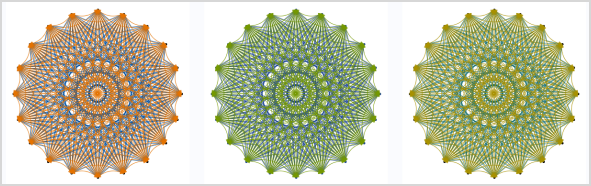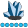## Advanced ODS Graphics techniques: new free book

#DataViz enthusiasts,

Our colleague @WarrenKuhfeld has recently published a free book titled, Advanced ODS Graphics Techniques.  The book is a free PDF download that brings together lots of documentation and examples from various sources, plus some great examples that put the techniques into practice.  I encourage you to read Warren's blog post to learn more and download the book.

I want to share one particularly cool example that I found in Chapter 5 of the book -- it reminds me of the Spirograph set I had as a child, but with neater output and less work.Here's Warren's example code ("Fun with Splines"), with minor adjustments from me, to create this visualization.  You can run it as-is in SAS Enterprise Guide with the HTML results turned on.  Or, you can modify it slightly (see the lines with notes in the comments) to run well in SAS University Edition or SAS Studio.  (And of course, you can specify your own ODS statements to run this in display manager/PC SAS).

``````/* Example from Chapter 5 of Warren Kuhfeld's book                                   */
/* Advanced ODS Graphics Examples                                                    */
data x(drop=t);
do id = 1 to 20;
t = (id - 1) * 2 * constant('pi') / 20;
x = cos(t);
y = sin(t);
output;
end;
run;

data curves(drop=id t: m d);
do id1 = 1 to 20;
do id2 = id1 + 1 to 20;
g + 1;
set x(rename=(x=t1 y=t2)) point=id1;
set x(rename=(x=t3 y=t4)) point=id2;
d = (t4 - t2) ** 2 + (t3 - t1) ** 2;
x1 = t1;
y1 = t2;
output; /* output the starting point */

td = ifn(abs(t4 - t2) lt 1e-12, 1e-12, t4 - t2);
m = -(t3 - t1) / td;
t1 = mean(t1, t3);
t2 = mean(t2, t4);
x1 = t1 + ifn(t1 gt 0 or td eq 1e-12, -1, 1) *
sqrt(0.1 * d / (1 + m * m));
y1 = m * (x1 - t1) + t2;
output; /* output the midpoint */

x1 = t3;
y1 = t4;
output; /* output the ending point */
end;
end;
stop;
run;

data both;
merge x curves;
run;

ods graphics / width=600 height=600 noborder;
/* Using ODS layout to align 3 graphs on one row */
ods layout start columns=3;

ods region;
/* When using EG with HTML output */
ods html(id=eghtml) style=plateau;
/* uncomment this for SAS Studio/SAS University Edition */
*ods html5(id=web) style=plateau;
proc sgplot data=both noautolegend noborder;
scatter y=y x=x / markerattrs=(symbol=circlefilled size=5px);
series y=y1 x=x1 / group=g lineattrs=graphdata1(pattern=solid);
spline y=y1 x=x1 / group=g lineattrs=graphdata2(pattern=solid)
xaxis display=none;
yaxis display=none;
run;

ods region;
/* When using EG with HTML output, comment */
ods html(id=eghtml) style=dove;
/* uncomment this for SAS Studio/SAS University Edition */
*ods html5(id=web) style=dove;
proc sgplot data=both noautolegend noborder;
scatter y=y x=x / markerattrs=(symbol=circlefilled size=5px);
series y=y1 x=x1 / group=g lineattrs=graphdata1(pattern=solid);
spline y=y1 x=x1 / group=g lineattrs=graphdata2(pattern=solid)
xaxis display=none;
yaxis display=none;
run;

ods region;
/* When using EG with HTML output */
ods html(id=eghtml) style=statdoc;
/* uncomment this for SAS Studio/SAS University Edition */
*ods html5(id=web) style=statdoc;
proc sgplot data=both noautolegend noborder;
scatter y=y x=x / markerattrs=(symbol=circlefilled size=5px);
series y=y1 x=x1 / group=g lineattrs=graphdata1(pattern=solid);
spline y=y1 x=x1 / group=g lineattrs=graphdata2(pattern=solid)
xaxis display=none;
yaxis display=none;
run;

ods layout end;``````
3 REPLIES 3

## Re: Advanced ODS Graphics techniques: new free book

Chris,

I have 9.4 TS Level 1M0 and it doesn't seem to recognize the Spline statement. Is there something comparable in my version?

Thanks,SuzanneDorinski
Lapis Lazuli | Level 10

## Re: Advanced ODS Graphics techniques: new free book

The SPLINE statement is new for SAS 9.4 maintenance release 3.  I currently have maintenance release 2 at work (TS1M2), so I can't generate the spirograph example yet either.

## Re: Advanced ODS Graphics techniques: new free book

I'm sorry that I missed that SPLINE is brand new for SAS 9.4m3.  You can play with this example if you use SAS Universty Edition: free software for learning SAS.  The current version uses SAS 9.4m3.  And no, you don't have to be a student to use this!  It's available for just about any non-commercial purpose.

Discussion stats
• 3 replies
• 3637 views
• 5 likes
• 3 in conversation